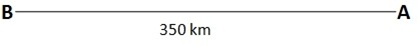### CAT 2018 – Slot 2 – Quantitative Ability – The value of the sum 7 x 11 + 11 x 15 + 15 x 19 + ...+ 95 x 99 is

Q. 1:The value of the sum 7 x 11 + 11 x 15 + 15 x 19 + …+ 95 x 99 is
1. 80707
2. 80773
3. 80730
4. 80751

7 x 11 + 11 x 15 + 15 x 19 + …+ 95 x 99

nth term of series = {4(n+1)-1}×{ 4 (n+1)+3}=16n^2+16+32n+8n+8-

3=16n^2+40n+21

Thus 7 x 11 + 11 x 15 + 15 x 19 + …+ 95 x 99 = ∑ Tn=∑ (16n^2+40n+15)

Required sum = ∑ Tn=∑ (16n^2+ 40n+15)=16 ×(n(n+1(2n+1))/6 + 40×n(n+1)/2 + 21n

Putting n= 23

Sum = 16×23×24×47/6+40×23×24/2+21×23

=69184+11040+483=80707

##### Q. 2: How many two-digit numbers, with a non-zero digit in the units place, are there which are more than thrice the number formed by interchanging the positions of its digits?1. 52. 63. 84. 7

Let ‘ab’ be the two digit number. Where b  0.

On interchanging the digits, the new number will be ‘ba’ As per the condition 10a+b > 3×(10b + a)

7a > 29b

For b = 1, a = {5, 6, 7, 8, 9}

For b = 2, a = {9}

For b = 3, no value of ‘a’ is possible. Hence, there are a total of 6 such numbers

Q. 3:The smallest integer n such that n^3 – 11n^2 + 32n – 28 > 0 is?

Given, n^3 – 11n^2 + 32n – 28 > 0

(n-7) (n-2)^2>0

Therefore n must be greater than 7.

So smallest integral value of n = 8

Q. 4: Gopal borrows Rs. X from Ankit at 8% annual interest. He then adds Rs. Y of his own money and lends Rs. X+Y to Ishan at 10% annual interest. At the end of the year, after returning Ankit’s dues, the net interest retained by Gopal is the same as that accrued to Ankit. On the other hand, had Gopal lent Rs. X+2Y to Ishan at 10%, then the net interest retained by him would have increased by Rs. 150. If all interests are compounded annually, then find the value of X + Y.

Interest to be repaid to Ankit at the end of the year = 0.08X

Interest that Gopal would receive from Ishan in two cases are as given. Case I: if he lends X + Y

Interest received = (X + Y) × 0.1 = 0.1X + 0.1Y Interest retained by Gopal after paying to Ankit

= (0.1X + 0.1Y) – (0.08X) = 0.02X + 0.1Y

Given that Interest retained by Gopal is same as that accrued by Ankit

=> (0.02X + 0.1Y) = 0.08X

=> Y = 0.6X

Case II: if he lends X + 2Y

Interest received = (X + 2Y) × 0.1 = 0.1X + 0.2Y Interest retained by Gopal after paying to Ankit

= (0.1X + 0.2Y) – (0.08X) = 0.02X + 0.2Y

Given that interest retained by Gopal would increase by 150

=> (0.02X + 0.2Y) – (0.02X + 0.1Y) = 150 0.1Y = 150

=> Y = 1500 and X = 1500×0.6= 2500 Hence X + Y = 2500 + 1500 = 4000

Q. 5: On a long stretch of east-west road, A and B are two points such that B is 350 km west of A. One car starts from A and another from B at the same time. If they move towards each other, then they meet after 1 hour. If they both move towards east, then they meet in 7 hrs. The difference between their speeds, in km per hour, isLet ‘x’ and ‘y’ be the speed (in km/hr) of cars starting from both A and B respectively. If they both move in east direction, then B will overtake A only if y > x.

Also, relative speed of both the cars when they move in east direction = (y – x) km/hr

It is mentioned that they take 7 hours to meet. i.e. they travel 350 km in 7 hours with a relative speed of (y-x) km/hr.

Hence, (y – x) = 350/7 = 50 km/hr.

##### Checkout Other Questions of CAT 2018 Slot 2 Paper:

Verbal Ability :              |   Q.01- Q.05  |  Q.06- Q.9  |  Q.10- Q.14  |  Q.15- Q.19  |  Q.20- Q.24  |  Q.25- Q.29  |  Q.30 – Q.34  |

Logical Reasoning :    |   Q.29 – Q.32  |

Quantitative Aptitude: |